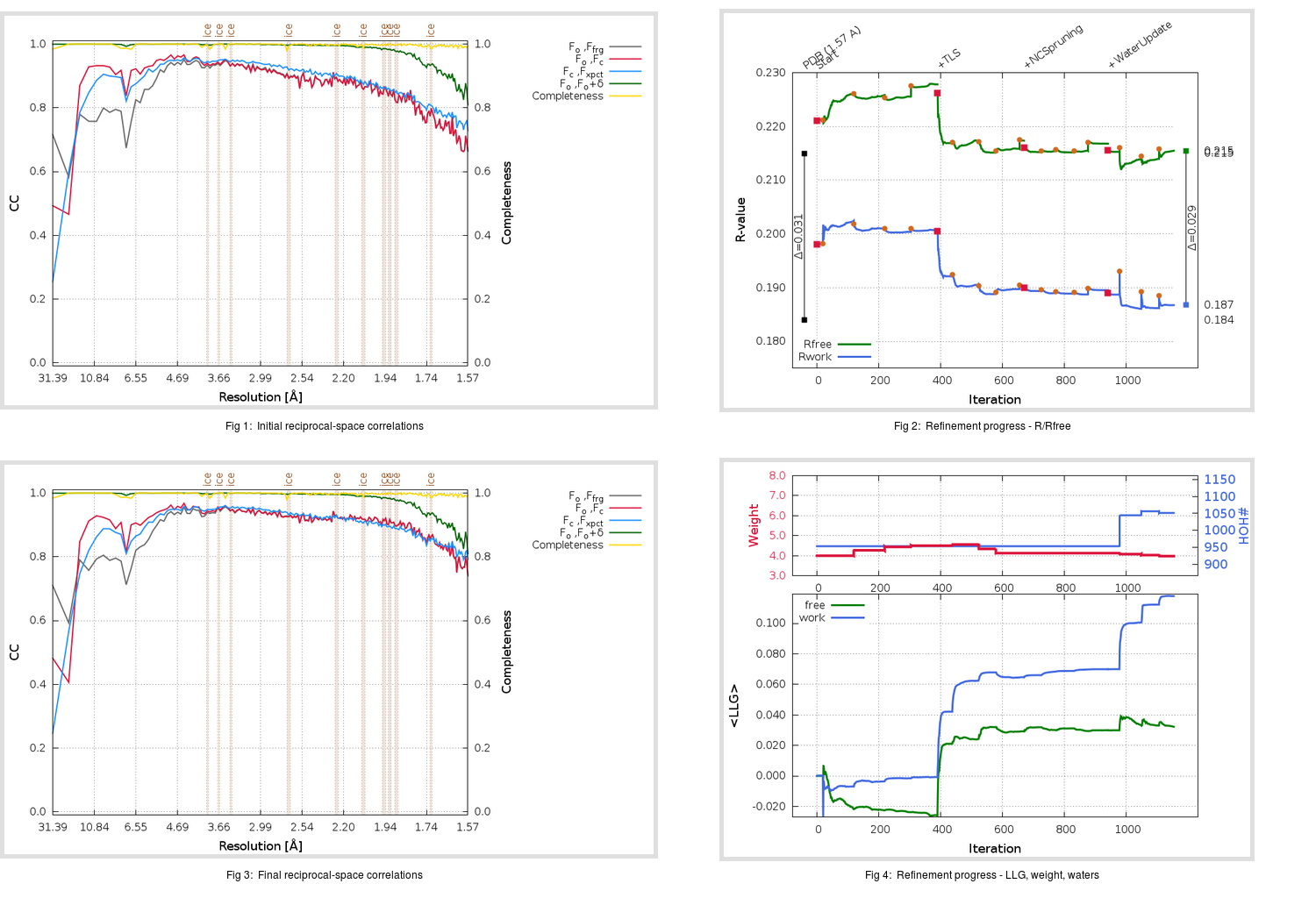Content:

```    Diffraction limits & principal axes of ellipsoid fitted to diffraction cut-off surface:
1.774         1.0000   0.0000   0.0000       a*
1.558         0.0000   1.0000   0.0000       b*
1.514         0.0000   0.0000   1.0000       c*
```

## Deposited

` `
 Date deposited Date data collection Resolution R, Rfree 20170529 20160227 1.57 0.1820 0.2150

Molprobity (CCP4 7.0 version) summary:

```Ramachandran outliers =   0.00 %
favored =  98.80 %
Rotamer outliers      =   0.67 %
C-beta deviations     =     0
Clashscore            =   2.55
RMS(bonds)            =   0.0063
RMS(angles)           =   1.18
MolProbity score      =   1.04
Resolution            =   1.57
R-work                =   0.1820
R-free                =   0.2150
```

```Number of waters      =   953

<B> (all atoms) =   27.42 ( sd =   12.76 ) for       7532 non-hydrogen atoms
<B>   (protein) =   25.80 ( sd =   12.24 ) for       6557 non-hydrogen atoms
<B>     (water) =   38.34 ( sd =   10.63 ) for        953 non-hydrogen atoms
<B>    (others) =   36.36 ( sd =   10.56 ) for         22 non-hydrogen atoms

B min/max       (all non-hydrogen atoms) =    9.29 /   85.14
B min/max   (protein non-hydrogen atoms) =    9.29 /   85.14
B min/max     (water non-hydrogen atoms) =   14.34 /   68.56
B min/max     (other non-hydrogen atoms) =   19.30 /   57.33
```

## BUSTER (re-)refinement

` `

Molprobity (CCP4 7.0 version) summary:

```Ramachandran outliers =   0.00 %
favored =  99.16 %
Rotamer outliers      =   0.93 %
C-beta deviations     =     0
Clashscore            =   1.70
RMS(bonds)            =   0.0110
RMS(angles)           =   1.61
MolProbity score      =   0.92
Resolution            =   1.57
R-work                =   0.1868
R-free                =   0.2155
```

```Number of waters      =  1051

<B> (all atoms) =   26.51 ( sd =   11.60 ) for       7630 non-hydrogen atoms
<B>   (protein) =   24.24 ( sd =    9.72 ) for       6557 non-hydrogen atoms
<B>     (water) =   40.59 ( sd =   12.42 ) for       1051 non-hydrogen atoms
<B>    (others) =   30.82 ( sd =    4.91 ) for         22 non-hydrogen atoms

B min/max       (all non-hydrogen atoms) =   10.76 /   78.43
B min/max   (protein non-hydrogen atoms) =   10.76 /   68.64
B min/max     (water non-hydrogen atoms) =   13.67 /   78.43
B min/max     (other non-hydrogen atoms) =   22.26 /   42.05
```

Refinement progression:Results:

` `
 File Remark 5VZR_aB_refine.01_04_refine.pdb.gz exact refinement commands are in header 5VZR_aB_refine.01_04_refine.mtz.gz including original deposited data and several re-refinement map coefficients 5VZR_aB_refine.01_04_BUSTER_model.cif.gz including any non-standard compound restraints 5VZR_aB_refine.01_04_BUSTER_refln.cif.gz